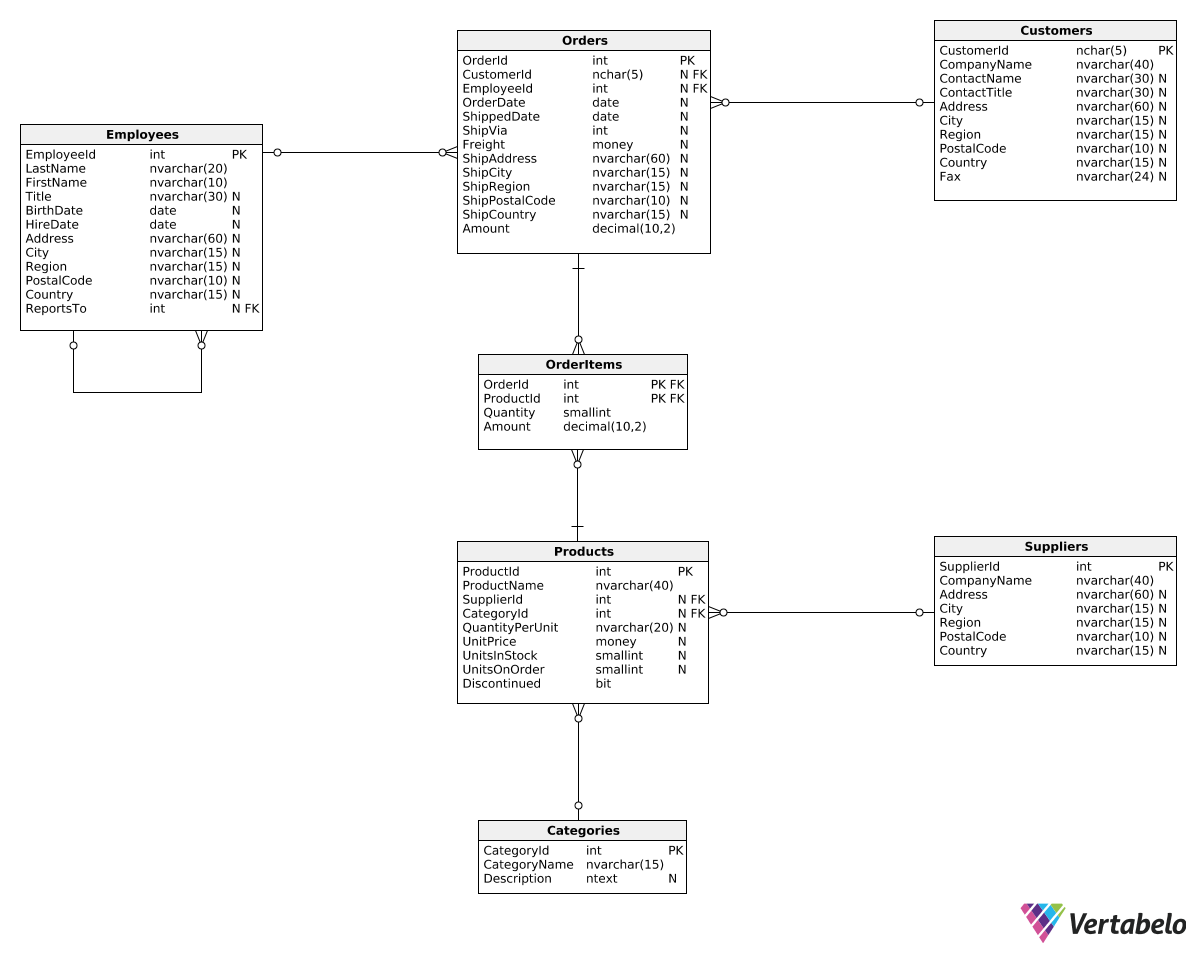Deals Of The Week - hours only!Up to 80% off on all courses and bundles.-Close
Introduction
Reports with revenue in categories
Revenue in time periods for selected categories
Summary

## Instruction

Good job! Now that we know how to add TotalRevenue as the last column, we can calculate the ratio between a category's revenue and total revenue. Such a report can help us quickly identify which categories generate the most revenue. Here's the query:

WITH AllCategories AS (
SELECT SUM(Amount) AS TotalSum
FROM Orders
)
SELECT
CategoryName,
SUM(OI.Amount) AS CategoryRevenue,
SUM(OI.Amount) / CAST(AC.TotalSum AS decimal(10,2)) AS TotalRevenueRatio
FROM AllCategories AC, OrderItems OI
JOIN Orders O
ON O.OrderId = OI.OrderId
JOIN Products P
ON OI.ProductId = P.ProductId
JOIN Categories C
ON P.CategoryId = C.CategoryId
GROUP BY CategoryName, AC.TotalSum;


In the third column, we now divide category revenue by total revenue and get the correct ratio. As usual, we're casting one of the values to a decimal type to avoid integer division.

## Exercise

Create a report of the category revenue for all orders placed in 2017 or later. Show three columns: CategoryName, CategoryRevenue, and TotalRevenuePercentage. The last column should show the rounded to two decimal places percentage of the total revenue generated by that category.

### Stuck? Here's a hint!

Filter rows in both the CTE and the outer query. To get percentages, multiply the ratio of category revenue to total revenue by 100.